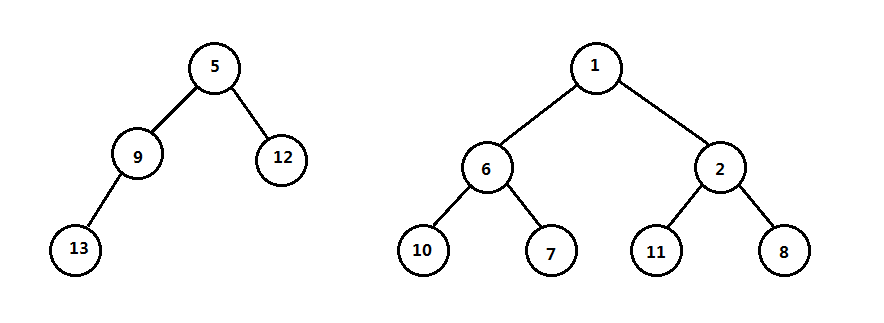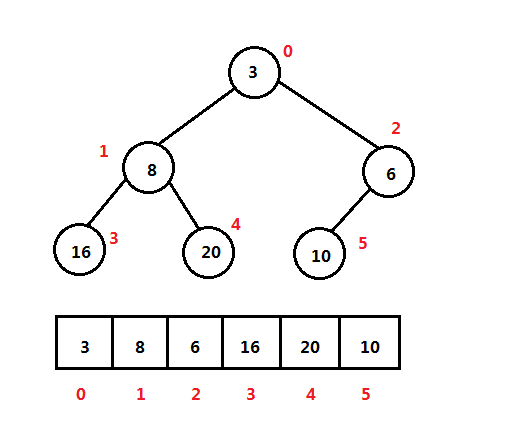# java优先队列出队,如何实现优先队列？Java使用数组实现最小堆和优先队列public interface TypeInterface {

// 获取权值或优先级

long getKey();

// 设置权值或优先级

void setKey(long key);

}

// Java实现优先队列，最小堆

public class PriorityQueue{

private int size;

private ArrayList array;

// 优先队列构造函数

public PriorityQueue() {

this.size = 0;

this.array = new ArrayList<>();

}

// 使用一个数组构建一个优先队列

public PriorityQueue(ArrayList extends T> list){

this.size = list.size();

this.array = new ArrayList<>(list);

}

// 检查优先队列是否已空

public boolean isEmpty(){

return this.size == 0;

}

// 获取父结点位置

private int parent(int i){

return (i - 1) / 2;

}

// 获取左儿子位置

private int left(int i){

return 2 * i + 1;

}

// 获取右儿子位置

private int right(int i){

return 2 * i + 2;

}

private void swap(int i, int j){

T temp = array.get(i);

array.set(i, array.get(j));

array.set(j, temp);

}

// 往队列中添加元素

public void push(T value){

if(value == null)

throw new NullPointerException();

this.size++;

int i = this.size - 1;

// 上滤

while(i != 0 && array.get(i).getKey() < array.get(parent(i)).getKey()){

swap(i, parent(i));

i = parent(i);

}

}

// 下滤

private void heapify(int i){

int left = left(i);

int right = right(i);

int small = i;

if(left < this.size && array.get(left).getKey() < array.get(i).getKey())

small = left;

if(right < this.size && array.get(right).getKey() < array.get(small).getKey())

small = right;

if(small != i){

swap(i, small);

heapify(small);

}

}

// 从堆顶取出一个元素

public T pop(){

if(this.isEmpty())

return null;

if(this.size == 1){

T v = array.get(0);

this.size--;

return v;

}

T value = array.get(0);

array.set(0, array.get(this.size - 1));

this.size--;

heapify(0);

return value;

}

// 根据数据索引降低关键字的值到value

public void decreaseKey(int i, long value){

if(this.isEmpty() || i >= this.size)

return;

array.get(i).setKey(value);

// 上滤

while(i != 0 && array.get(i).getKey() < array.get(parent(i)).getKey()){

swap(i, parent(i));

i = parent(i);

}

}

// 根据索引删除一个数据

public void delete(int i){

if(this.isEmpty() || i >= this.size)

return;

this.decreaseKey(i, Integer.MIN_VALUE);

this.pop();

}

// 快速构建一个堆

public void buildHeap(){

if(this.isEmpty())

return;

for(int i = this.size / 2;i >= 0;i--)

heapify(i);

}

}

public class Lengua implements TypeInterface{

private long id;

private String name;

public Lengua(long id, String name) {

this.id = id;

this.name = name;

}

@Override

public long getKey() {

return this.id;

}

@Override

public void setKey(long key) {

this.id = key;

}

public long getId() {

return id;

}

public void setId(long id) {

this.id = id;

}

public String getName() {

return name;

}

public void setName(String name) {

this.name = name;

}

}

// 例子1

PriorityQueue heap = new PriorityQueue<>();

Lengua espanol = new Lengua(90, "espanol");

Lengua chino = new Lengua(50, "chino");

Lengua italiano = new Lengua(48, "italiano");

Lengua frances = new Lengua(31, "frances");

Lengua aleman = new Lengua(29, "aleman");

heap.push(espanol);

heap.push(chino);

heap.push(italiano);

heap.push(frances);

heap.push(aleman);

heap.decreaseKey(3, 16);

while (!heap.isEmpty()){

Lengua lengua = heap.pop();

System.out.println(lengua.getId() + " " + lengua.getName());

}

System.out.println();

// 例子2

ArrayList list = new ArrayList<>();